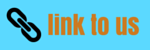# Thermal Properties of Matter

## 6. Thermal stress

• If we clamp the ends of a rod rigidly to prevent expansion or contraction and then change the temperature, tensile or compressive stresses called thermal stresses develop.
• The rod would like to expand or contract, but the clamps won't let it. The resulting stresses may become large enough to strain the rod irreversibly or even break it.
• Engineers must account for thermal stress when designing structures. Concrete highways and bridge decks usually have gaps between sections, filled with a flexible material or bridged by interlocking teeth , to permit expansion and contraction of the concrete.
• To calculate the thermal stress in a clamped rod, we compute the amount the rod would expand (or contract) if not held and then find the stress needed to compress (or stretch) it back to its original length. Suppose that a rod with length $L$ and cross-sectional area $A$ is held at constant length while the temperature is reduced (negative $\Delta T$), causing a tensile stress. The fractional change in length if the rod were free to contract would be
$\left ( \frac{\Delta L}{L} \right )_{thermal}=\alpha \Delta T$
Here both $\Delta L$ and $\Delta T$ are negative.The tension must increase by an amount F that is just enough to produce an equal and opposite fractional change in length $\left ( \frac{\Delta L}{L} \right )_{tension}$. From the definition of Young's modulus,
$Y=\frac{F/A}{\Delta L /L}$
or,
$\left ( \frac{\Delta L}{L} \right )_{tension}=\frac{F}{AY}$
If the length is to be constant, the total fractional change in length must be zero. This means that
$\left ( \frac{\Delta L}{L} \right )_{thermal}+\left ( \frac{\Delta L}{L} \right )_{tension} = \alpha \Delta T + \frac{F}{AY} = 0$
Solving for the tensile stress $F/A$ required to keep the rod's length constant, we find
\begin{equation} \frac{F}{A}=-Y\alpha \Delta T \tag{7} \end{equation} For a decrease in temperature, $\Delta T$ is negative, so $F$ and $F/A$ are positive; this means that a tensile force and stress are needed to maintain the length. If $\Delta T$ is positive, $F$ and $F/A$ are negative, and the required force and stress are compressive.

### 7. Specific Heat Capacity

• If a system undergoes a change of temperature from T to T+ΔT during the transfer of ΔQ amount of heat then heat capacity c of the system is defined as the ratio of

$$c=\frac{\Delta Q}{\Delta T}$$
• Thus Heat capacity per unit mass of a substance is its specific heat capacity. $$c=\frac{\Delta Q}{m\Delta T}$$

where,
m - mass of the substance
ΔQ - Heat absorbed or rejected by the substance
ΔT - Change in Temperature

• Specific heat capacity depends on the nature of substance.
• It is constant characterstics of the substance and is independent of the ammount of substance.
• It also depends on the temperature of the substance
• Its unit is J Kg-1 K-1
• If the amount of substance is specified in terms of no of moles n instead of mass m then the heat capacity per mole of the substance is

$$=\frac{\Delta Q}{n\Delta T}$$

and is known as Molar Specific Heat capacity.

• It is constant characterstics of the substance and independent of the ammount of substance
• It depends on the nature of the substance ,temperature and amount of heat supplied
• Its unit is J mol-1 K-1
• In case of gases , when a gas is heated, ordinarly there is change in volume as well as pressure in addition to change in temperature
• For simiplicity either volume or pressure can be kept constant.Thus gas have two specific heat capacities
1) Specific heat capacity at constant volume CV
2) Specific heat capacity at constant pressure CP